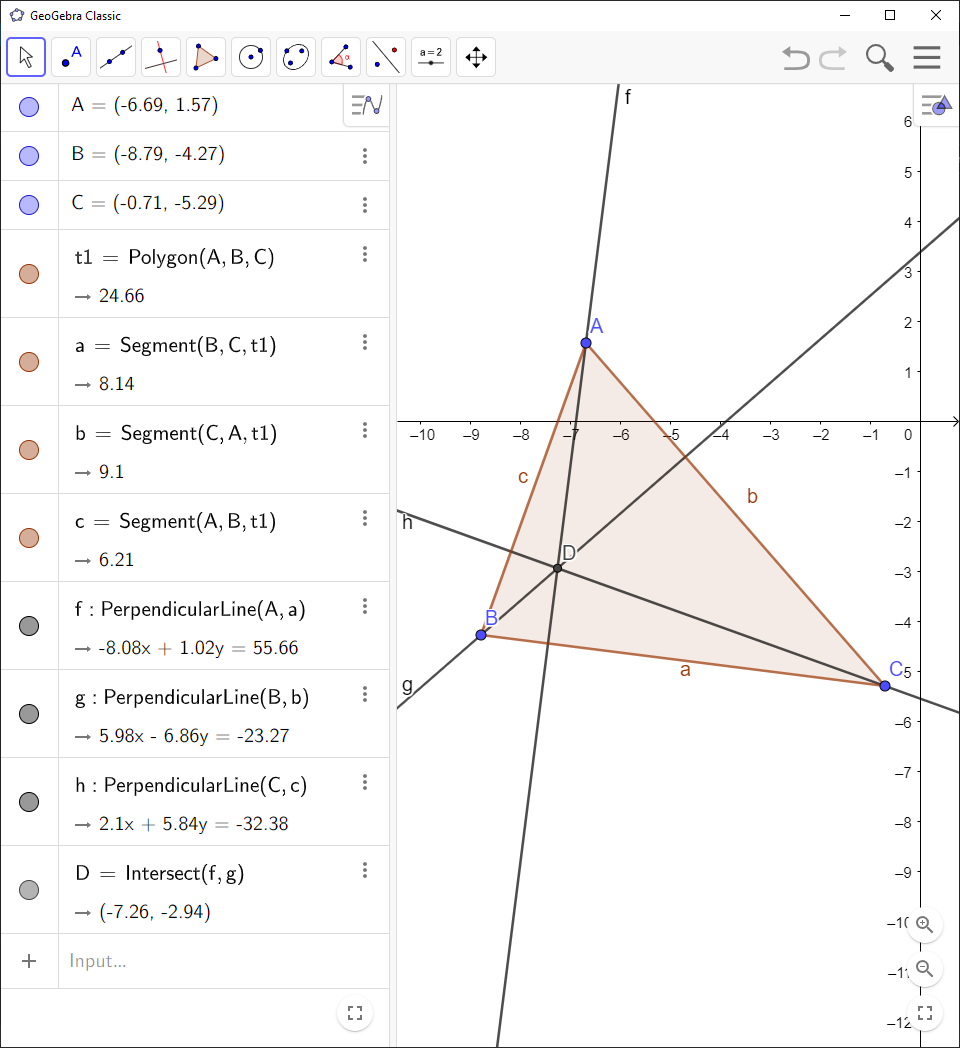# How to Find Orthocenter and Altitudes with GeoGebra

You can use `GeoGebra` to find the orthocenter and altitudes of a triangle:

`GeoGebra` Instruction 1

1.
Open `Algebra View` and `Graphics View` under`View` in`Menu`.
2.
Make a triangle with the vertices $A$, $B$ and $C$ by clicking `Polygon`. Then you need to click on three positions in `Graphics View` where you want the vertices of the triangle to be. Finally, complete the triangle by clicking on the point that you started with.
3.
Select `Perpendicular Line`. Construct the line from vertex $A$ perpendicular to the opposite side $BC$, construct the line from vertex $B$ perpendicular to the opposite side $AC$, and construct the line from vertex $C$ perpendicular to the opposite side $AB$. These lines are the altitudes.
4.
Select `Intersect`by first clicking tool number 2, then tool number 4 in the drop-down list, and click on two of the altitudes to construct the point of intersection between them. This point is the orthocenter.